# Pynbody Demo¶

This notebook highlights some of the basic pynbody functionality to get you on the way to analyzing your own simulations. The pynbody webpage is here and you can find the documentation with additional tutorials here. If you find that things are broken please please please let us know by submitting a bug report on github. If you want to run the notebook on your own machine, you need to get the testdata tarball and change the path in the load function below accordingly.

In :
%matplotlib inline
from matplotlib.pylab import *
rcParams['figure.figsize'] = (10,6)
rcParams['font.size'] = 18


loading a simulation output using the pynbody.load() function, which tries to automatically determine which type of code output you have:

In :
import pynbody


Note that the above assumes you have downloaded and unpacked the test data (in the nose folder). You can obtain it here, if you haven't already. Or, of course, you can just substitute your favourite cosmological simulation. A quick look at some basic information about the run we just opened:

In :
s

Out:
<SimSnap "../nose/testdata/g15784.lr.01024" len=1717156>
In :
len(s)

Out:
1717156
In :
len(s.stars)

Out:
265170

stars, gas, dark also available as s, g, d

In :
len(s.g), len(s.gas), len(s.dark)

Out:
(158755, 158755, 1293231)

the properties attribute of a SimSnap tells us some more basic info

In :
s.properties

Out:
{'omegaM0': 0.24,
'omegaL0': 0.76,
'h': 0.7301145776501103,
'boxsize': Unit("6.85e+04 kpc a"),
'a': 0.9999999999999911,
'time': Unit("1.40e+01 s kpc km**-1")}
In :
s.properties['time'].in_units('Gyr')

Out:
13.72831064485079

which quantities do we have available?

In :
s.keys()

Out:
[]

None! Because pynbody "lazy-loads" data... so lets see which data is actually on-disk:

In :
s.loadable_keys()

Out:
['mass',
'eps',
'HeI',
'HeII',
'igasorder',
'pos',
'OxMassFrac',
'coolontime',
'array_write_test',
'vel',
'iord',
'HI',
'FeMassFrac',
'phi']

## Accessing data¶

to access any of these arrays or vectors, you access them like a python dictionary:

In :
s['pos'].in_units('kpc')

Out:
SimArray([[   733.51516346,  -2479.31184326, -11394.52608302],
[   781.21390409,  -2209.05638147, -11392.16434933],
[   794.4949563 ,  -2232.21137376, -11432.30055384],
...,
[  1672.78285106,  -2332.06734179,  -8386.4632496 ],
[  1675.12072757,  -2335.55559387,  -8386.09837501],
[  1682.45100698,  -2338.79940549,  -8386.07898308]], 'kpc')

Note that each array has units attached...

In :
s['mass'], s['vel'], s.g['HeI'], s.s['posform']

No H2 data found in StarLog file

Out:
(SimArray([3.19310585e-11, 3.19310585e-11, 3.19310585e-11, ...,
1.24175999e-11, 1.24175999e-11, 1.24175999e-11], '4.75e+16 Msol'),
SimArray([[ 0.01673503, -0.01547551, -0.16838804],
[ 0.05142973, -0.00647544, -0.16129912],
[ 0.02738659, -0.00184103, -0.1736342 ],
...,
[-0.13633673, -0.13716312, -0.12805983],
[-0.13267632, -0.09228273, -0.1247804 ],
[ 0.06591902,  0.13406622, -0.15172358]], '1.73e+03 km a s**-1'),
SimArray([3.5166965e-12, 1.6011119e-09, 1.8811888e-10, ..., 1.9425629e-12,
2.3049744e-12, 1.8176783e-11], dtype=float32, '1.00e+00'),
SimArray([[ 0.0178572 , -0.0131114 , -0.00092187],
[ 0.01790398, -0.01335163, -0.00124265],
[ 0.01781229, -0.01359182, -0.00148088],
...,
[ 0.02442261, -0.03404816, -0.12244228],
[ 0.02445674, -0.03409909, -0.12243695],
[ 0.02456377, -0.03414645, -0.12243667]], '6.85e+04 kpc aform'))

by default everything is in system units, but most of the time thinking in physical units is easier:

In :
s.physical_units()


We have defined many useful quantities that are automatically calculated for you. For example, the radial and tangential velocities are simply obtained by

In :
s['vt'],s['vr']

Out:
(SimArray([ 37.28290565,  81.78390831,  60.75947459, ..., 330.09191502,
288.75911925, 303.1394157 ], 'km s**-1'),
SimArray([291.20028176, 281.05583586, 297.57331009, ..., 227.22613725,
202.62874018, 208.42590924], 'km s**-1'))

you can get a list of all available derivable quantities

In :
s.derivable_keys()[0:10]

Out:
['HII',
'HeIII',
'ne',
'hetot',
'hydrogen',
'feh',
'oxh',
'ofe',
'mgfe',
'nefe']

if we have information about substructure (i.e. from the Amiga Halo Finder) we can load in the halos:

In :
h = s.halos()


We are not really near the main halo, so lets center the simulation there using the "shrinking sphere" method

In :
pynbody.analysis.halo.center(h,mode='ssc')

Out:
<pynbody.transformation.GenericTranslation at 0x7fadb1033b20>

we're going to work with the main halo a lot, so it's useful to save a reference to it in a variable to save some typing:

In :
h1 = h
h1

Out:
<SimSnap "../nose/testdata/g15784.lr.01024:halo_1" len=502300>

this new object is a SubSnap and behaves exactly like any other SimSnap:

In :
len(h1.g), len(h1.s)

Out:
(79060, 262178)
In :
h['mass'].sum().in_units('1e12 Msol')

Out:
SimArray(1.69639241, '1.00e+12 Msol')

## Rendering images¶

lets look at a picture of the dark matter distribution of a part of the whole simulation...

In :
im = pynbody.plot.image(s.d,width='50 Mpc', units='Msol kpc^-2', cmap=cm.Greys)

/opt/anaconda3/lib/python3.8/site-packages/matplotlib/colors.py:1173: RuntimeWarning: overflow encountered in log
np.log(resdat, resdat)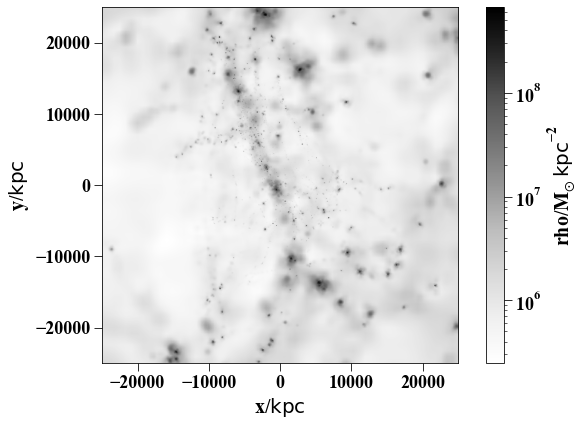In :
pynbody.plot.image(h.g,width='200 kpc', av_z=True, cmap=cm.Blues)

Out:
SimArray([[777.3692 , 779.5763 , 781.7839 , ..., 619.7823 , 617.5206 ,
615.2592 ],
[780.5642 , 782.8128 , 785.06195, ..., 621.82996, 619.56494,
617.3005 ],
[783.75726, 786.0473 , 788.33795, ..., 623.8763 , 621.608  ,
619.3404 ],
...,
[740.93774, 743.1989 , 745.4564 , ..., 888.4307 , 885.5547 ,
882.67755],
[737.3295 , 739.5767 , 741.8202 , ..., 886.3587 , 883.51935,
880.67957],
[733.7115 , 735.94495, 738.17456, ..., 884.28467, 881.4824 ,
878.6798 ]], dtype=float32, 'Msol kpc**-3')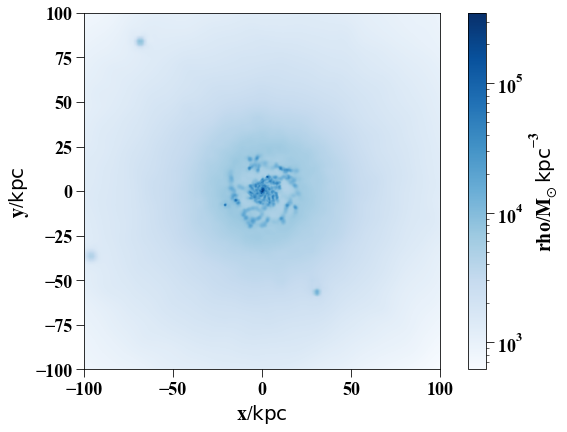Typically we want to make sure the system is aligned in some predictable way, so pynbody allows us to easily make the disk angular momentum axis the z-axis:

In :
pynbody.analysis.angmom.faceon(h,cen=(0,0,0))

Out:
<pynbody.transformation.GenericRotation at 0x7fadb1dbd370>

we use cen=(0,0,0) because we have already centered it in the previous step... if you don't specify a center, it is first centered and then aligned. Now lets look at a slice of the gas disk edge-on:

In :
s.rotate_x(90)
pynbody.plot.image(h.g,width='50 kpc',cmap=cm.Blues)

Out:
SimArray([[19602.258, 19579.627, 19556.992, ..., 20811.404, 20849.715,
20888.027],
[19652.078, 19631.777, 19611.475, ..., 20966.773, 21006.59 ,
21046.406],
[19701.898, 19683.926, 19665.957, ..., 21122.14 , 21163.465,
21204.787],
...,
[23214.576, 23186.46 , 23158.34 , ..., 19976.223, 19983.758,
19991.295],
[23105.31 , 23080.627, 23055.941, ..., 19893.758, 19896.078,
19898.398],
[22996.041, 22974.791, 22953.543, ..., 19811.297, 19808.398,
19805.5  ]], dtype=float32, 'Msol kpc**-3')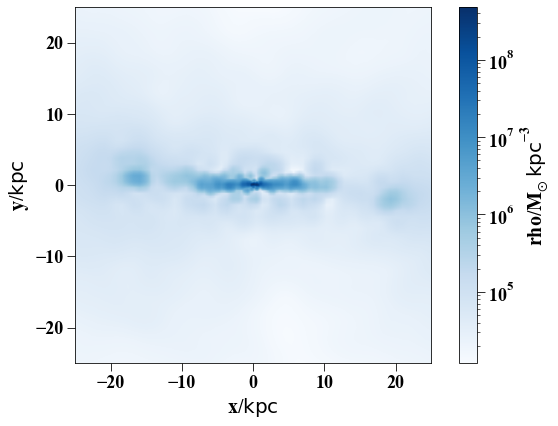By default, rho (i.e. density) is used to render the image, but you can any valid array is fair game:

In :
pynbody.plot.image(h1.g,qty='temp',width='40 kpc', cmap=cm.YlOrRd)
s.rotate_x(-90)

Out:
<pynbody.transformation.GenericRotation at 0x7fada277f3d0>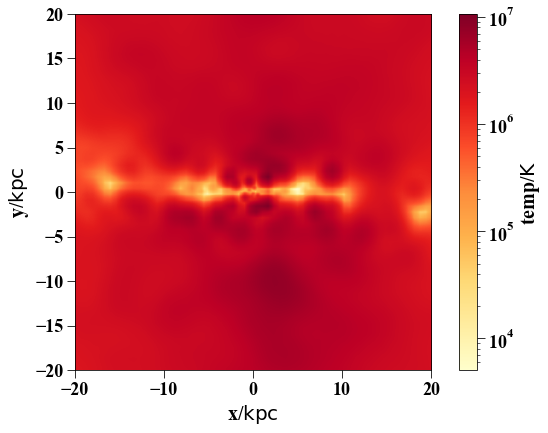## Profiles¶

In :
p = pynbody.analysis.profile.Profile(h1,max='200 kpc',min='.1 kpc',type='log',nbins=100)
ps = pynbody.analysis.profile.Profile(h1.s,max='50 kpc',nbins=50)
pg = pynbody.analysis.profile.Profile(h1.g,max='50 kpc',nbins=50)
plot(p['rbins'], p['density'], label = 'all')
plot(ps['rbins'], ps['density'], label = 'stars')
plot(pg['rbins'], pg['density'], label = 'gas')
semilogy()
xlim(0,50)
legend()
xlabel('$R$ [kpc]')
ylabel('$\Sigma$ [M$_{\odot}$ kpc$^{-2}$]')

/Users/app/Science/pynbody/pynbody/array.py:865: FutureWarning: Using a non-tuple sequence for multidimensional indexing is deprecated; use arr[tuple(seq)] instead of arr[seq]. In the future this will be interpreted as an array index, arr[np.array(seq)], which will result either in an error or a different result.
return self.base[self._reexpress_index(item)]

Out:
Text(0, 0.5, '$\\Sigma$ [M$_{\\odot}$ kpc$^{-2}$]')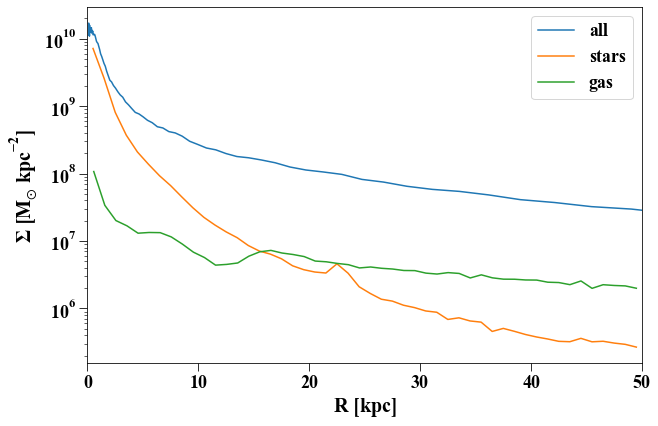The Profile class has lots of built-in functionality... including calculating a rotation curve:

In :
plot(p['rbins'], p['v_circ'], label = 'all')
plot(ps['rbins'], ps['v_circ'], label = 'stars')
plot(pg['rbins'], pg['v_circ'], label = 'gas')
xlim(0,50)
xlabel('$R$ [kpc]')
ylabel('$V_{circ}$')
legend()

pynbody.analysis.profile : Profile v_circ -- this routine assumes the disk is in the x-y plane
pynbody.analysis.profile : Profile v_circ -- this routine assumes the disk is in the x-y plane
pynbody.analysis.profile : Profile v_circ -- this routine assumes the disk is in the x-y plane

Out:
<matplotlib.legend.Legend at 0x7fada101fac0>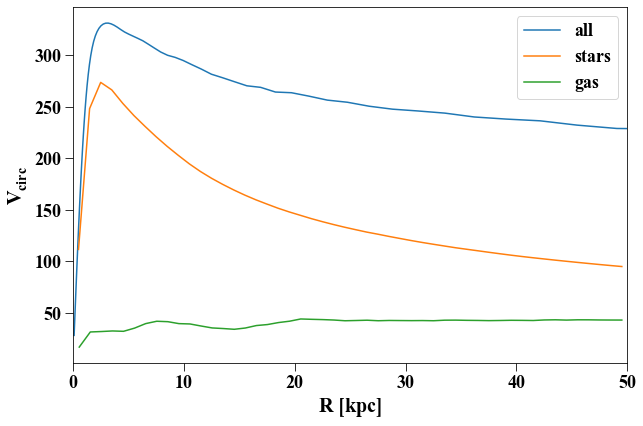Profile can use any loaded (or loadable/derivable!) array:

In :
plot(ps['rbins'],ps['age'])
xlabel('$R$ [kpc]')
ylabel('Age [Gyr]')

Out:
Text(0, 0.5, 'Age [Gyr]')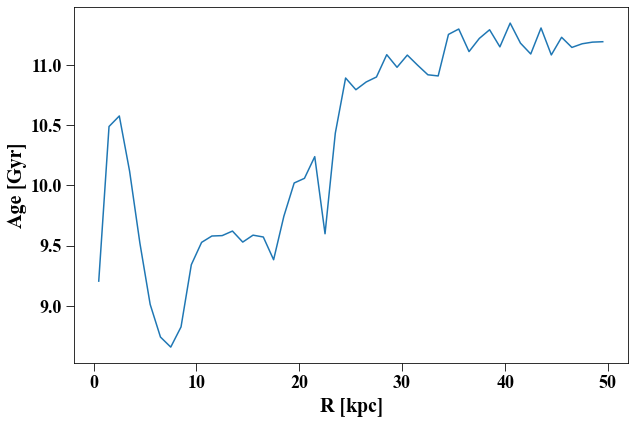By default, Profile calculates the means of quantities, but it trivially calculate derivatives and root-mean-square values as well:

In :
plot(ps['rbins'],ps['d_v_circ'])
xlabel('$R$ [kpc]')
ylabel('d$v_{c}$/d$R$')

Out:
Text(0, 0.5, 'd$v_{c}$/d$R$')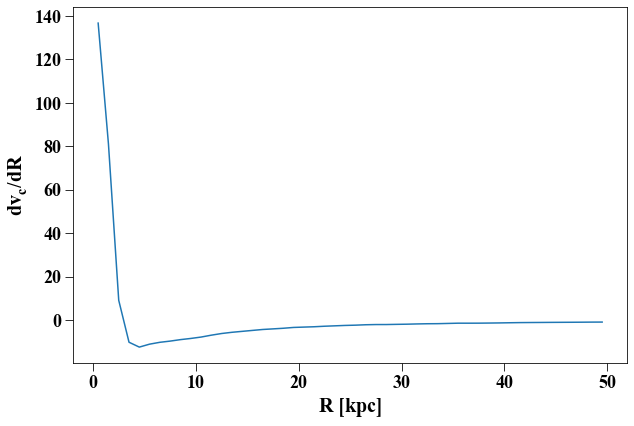In :
plot(ps['rbins'],ps['vr_rms'])
xlabel('R [kpc]')
ylabel('$v_{rms}$')

Out:
Text(0, 0.5, '$v_{rms}$')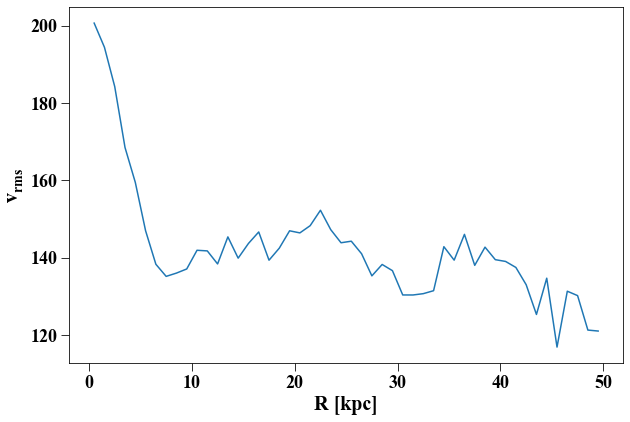## Filters¶

You can define filters to easily select particles based on their quantities. For example, to get stars that are 1-2 Gyr old:

In :
agefilt = pynbody.filt.BandPass('age', '1 Gyr','2 Gyr')
agefilt

Out:
BandPass('age', 'Gyr', '2.00e+00 Gyr')
In :
sphere = pynbody.filt.Sphere('50 kpc')
h1[sphere].s

Out:
<SimSnap "../nose/testdata/g15784.lr.01024:halo_1:sphere::star" len=242858>
In :
h1.s[sphere]['mass'].sum().in_units('1e12 Msol')

Out:
SimArray(0.10313312, '1.00e+12 Msol')

you can combine filters by using simple logic operators -- so to get the total mass of all 1-2 Gyr old stars within 50 kpc, you can do

In :
h1.s[sphere&agefilt]['mass'].sum().in_units('1e12 Msol')

Out:
SimArray(0.00231785, '1.00e+12 Msol')

## Units¶

You've noticed by now that we've been asking for things in various kinds of units. Pynbody has a unit-handling system built in that automatically ensures that arrays in different units are compatible and performs the necessary conversions for calculations. Adding mass and position together makes no sense, and pynbody knows this:

In :
s['mass'] + s['x']

---------------------------------------------------------------------------
UnitsException                            Traceback (most recent call last)
~/Science/pynbody/pynbody/units.py in ratio(self, other, **substitutions)
248         try:
--> 249             return (self / other).dimensionless_constant(**substitutions)
250         except UnitsException:

~/Science/pynbody/pynbody/units.py in dimensionless_constant(self, **substitutions)
545             else:
--> 546                 raise UnitsException("Not dimensionless")
547

UnitsException: Not dimensionless

During handling of the above exception, another exception occurred:

UnitsException                            Traceback (most recent call last)
399             try:
--> 400                 cr = x.units.ratio(self.units,
401                                    **context)

~/Science/pynbody/pynbody/units.py in ratio(self, other, **substitutions)
250         except UnitsException:
--> 251             raise UnitsException("Not convertible")
252

UnitsException: Not convertible

During handling of the above exception, another exception occurred:

ValueError                                Traceback (most recent call last)
<ipython-input-35-68f62327cbbe> in <module>
----> 1 s['mass'] + s['x']
2

~/Science/pynbody/pynbody/array.py in __add__(self, x)
432             return x + self
433         else:
--> 434             return self._generic_add(x)
435
436     def __sub__(self, x):

401                                    **context)
402             except units.UnitsException:
--> 403                 raise ValueError("Incompatible physical dimensions %r and %r, context %r" % (
404                     str(self.units), str(x.units), str(self.conversion_context())))
405

ValueError: Incompatible physical dimensions 'Msol' and 'kpc', context "{'a': 0.9999999999999911, 'h': 0.7301145776501103}"

but, it handles this just fine and returns an array with the right units:

In [ ]:
s['mass']*s['x']


This concludes the basic overview of what pynbody can do -- for more tutorials and in-depth explanation of the inner-workings of the framework, head over to the walkthroughs and cookbook examples. If you're stuck implementing a particular analysis workflow, stop by our Github issues and post your question, we'll be happy to help.